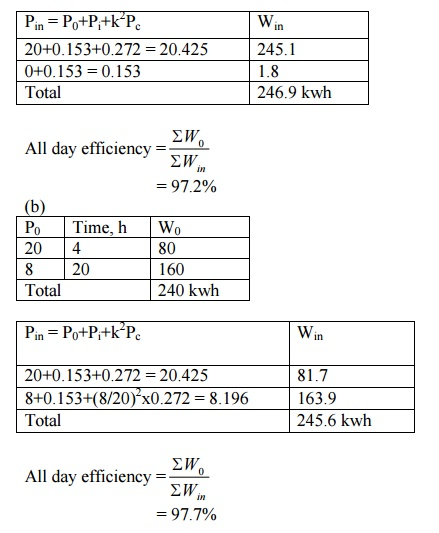Home | | Electrical Engineering and Instrumentation | Solved Problems: Electrical Engineering and Instrumentation - Transformer

# Solved Problems: Electrical Engineering and Instrumentation - Transformer

Electrical Engineering and Instrumentation - Transformer - Solved Problems: Electrical Engineering and Instrumentation - Transformer

PROBLEMS

A 400 kVA transformer has a primary winding resistance of 0.5 ohm and a secondary winding resistance of 0.001 ohm. The iron loss is 2.5 Kw and the primary and secondary voltages are 5 kV and 320 V respectively. If the power factor of the load is 0.85, determine the efficiency of the transformer (i) on full load and (ii) on half load.

Solution

Rated output = 400 kVA = 400x103 kVA

Full load secondary current, I2 = Rated output/V2 = 1250 A

Total resistance referred to secondary, re2 = r2+r1(V2/V1)2 = 0.033 ohm Full load copper loss, Pc = I22 re2 = 51.5625 Kw

Iron loss, Pi = 2.5 x103 watts

(i) Transformer efficiency at full load and 0.85 pf= 86.2%

(ii) Transformer efficiency at half load and 0.85 pf= 91.69%

2. Find all day efficiency of a transformer having maximum efficiency of 98% at 15 kVA at unity power factor. Compare its all day efficiencies for the following load cycles:

a. Full load of 20 kVA, 12 hours per day and no load rest of the day. b. Full load, 4 hours per day and 0.4 full load rest of the day.

Assume the load to operate on upf all day

SolutionStudy Material, Lecturing Notes, Assignment, Reference, Wiki description explanation, brief detail
Electrical Engineering and Instrumentation : Transformer : Solved Problems: Electrical Engineering and Instrumentation - Transformer |